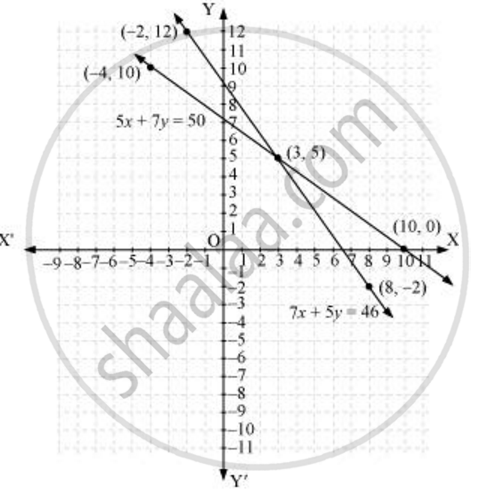# Form the pair of linear equations in the following problems, and find their solutions graphically 5 pencils and 7 pens together cost Rs 50, whereas 7 pencils and 5 pens together cost Rs 46. Find the cost of one pencil and that of one pen - Mathematics

Form the pair of linear equations in the following problems, and find their solutions graphically

5 pencils and 7 pens together cost Rs 50, whereas 7 pencils and 5 pens together cost Rs 46. Find the cost of one pencil and that of one pen

#### Solution

Let the cost of 1 pencil be Rs x and the cost of 1 pen be Rs y.

According to the question, the algebraic representation is

5x + 7y = 50

7x + 5y = 46

For 5x + 7y = 50,

x = (50 - 7y)/5

 x 3 10 -4 y 5 0 10

7x + 5y = 46

x = (46-5y)/7

Hence, the graphic representation is as follows.From the figure, it can be observed that these lines intersect each other at point (3, 5). Therefore, the cost of a pencil and a pen are Rs 3 and Rs 5 respectively

Concept: Graphical Method of Solution of a Pair of Linear Equations
Is there an error in this question or solution?

#### APPEARS IN

NCERT Class 10 Maths
Chapter 3 Pair of Linear Equations in Two Variables
Exercise 3.2 | Q 1.2 | Page 49
RD Sharma Class 10 Maths
Chapter 3 Pair of Linear Equations in Two Variables
Exercise 3.2 | Q 34.2 | Page 31